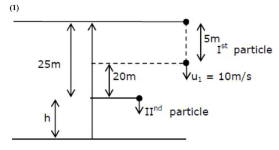# A stone is dropped from the top of a building.Question:

A stone is dropped from the top of a building. When it crosses a point $5 \mathrm{~m}$ below the top, another stone starts to fall from a point $25 \mathrm{~m}$ below the top, Both stones reach the bottom of building simultaneously. The height of the building is:

1. (1) $45 \mathrm{~m}$

2. (2) $35 \mathrm{~m}$

3. (3) $25 \mathrm{~m}$

4. (4) $50 \mathrm{~m}$

Correct Option: 1

Solution:For particle (1) $20+h=10 t+\frac{1}{2} g t^{2} \quad \ldots . .(i)$

For particle (2) $h=\frac{1}{2} \mathrm{~g} t^{2} \quad \ldots$ (ii)

put equation (ii) in equation (i)

$20+\frac{1}{2} g t^{2}=10 t+\frac{1}{2} g t^{2}$

$t=2$ sec

Put in equation (ii)

$h=\frac{1}{2} g t^{2}$

$=\frac{1}{2} \times 10 \times 2^{2}$

$h=20 \mathrm{~m}$

the height of the building $=25+20=45 \mathrm{~m}$